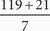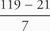# SAT Math Multiple Choice Question 78: Answer and Explanation

### Test Information

Question: 78

3. Sai is ordering new shelving units for his store. Each unit is 7 feet in length and extends from floor to ceiling. Sai's store has 119 feet of wall space that includes 21 feet of windows along the walls. If the shelving units cannot be placed in front of the windows, which of the following inequalities includes all possible values of r, the number of shelving units that Sai could use?

• A. r• B. r• C. r ≤ 119 - 21 + 7r
• D. r ≥ 119 + 21 - 7r

A To figure out the total number of shelving units Sai could use, find the total available wall space and divide by the length of the units. The total amount of wall space can be calculated as 119 - 21. Because the length of each unit is 7 feet, the maximum number of units Sai could put up can be calculated as. Because this is the maximum number of units Sai could put up, r has to be less than or equal to this number. Therefore, the correct answer is (A).